Share

# Balbharati solutions for Maharashtra state board SSC 9th Standard Science and Technology chapter 3 - Current Electricity [Latest edition]

Textbook page

#### Chapters## Chapter 3: Current Electricity

Exercise

### Balbharati solutions for Maharashtra state board SSC 9th Standard Science and Technology Chapter 3 Current Electricity Exercise [Pages 44 - 45]

Exercise | Q 1 | Page 44

The accompanying figure shows some electrical appliances connected in a circuit in a house. Answer the following questions.

A. By which method are the appliances connected?
B. What must be the potential difference across individual appliances?
C. Will the current passing through each appliance be the same? Justify your answer.
D. Why are the domestic appliances connected in this way?
E. If the T.V. stops working, will the other appliances also stop working? Explain your answer.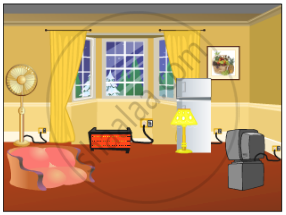Exercise | Q 2 | Page 44

The following figure shows the symbols for components used in the accompanying electrical circuit. Place them at proper places and complete the circuit.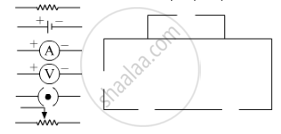Which law can you prove with the help of above circuit ?

Exercise | Q 3 | Page 44

Umesh has two bulbs having resistances of 15 W and 30 W.  He wants to connect them in a circuit, but if he connects them one at a time the filament gets burnt. Answer the following.

A.Which method should he use to connect the bulbs?
B. What are the characteristics of this  way of connecting the bulbs depending on the answer of A above
C . What will be the effective resistance in the above circuit?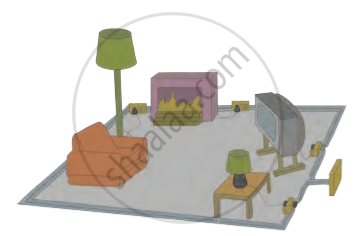Exercise | Q 4.1 | Page 44

The following table shows current in Amperes and potential difference in Volts.
Find the average resistance.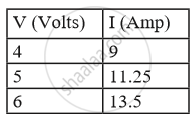Exercise | Q 4.2 | Page 44

The following table shows current in Amperes and potential difference in Volts.
What will be the nature of the graph between the current and potential difference? (Do not draw a graph.)Exercise | Q 4.3 | Page 44

The following table shows current in Amperes and potential difference in Volts.
Which law will the graph prove? Explain the law.Exercise | Q 5 | Page 44

Match the pairs

 ‘A’ Group ‘B’ Group 1.Free electrons a. V/ R 2. Current b. Increases the     resistance in the     circuit 3. Resistivity c. Weakly attached 4. Resistances in series d. VA/LI
Exercise | Q 6 | Page 45

The resistance of a conductor of length x is r. If its area of crosssection is a, what is its resistivity? What is its unit?

Exercise | Q 7 | Page 45

Resistances R1, R2, R3 and R4 are connected as shown in the figure. S1 and S2 are two keys. Discuss the current flowing in the circuit in the following cases.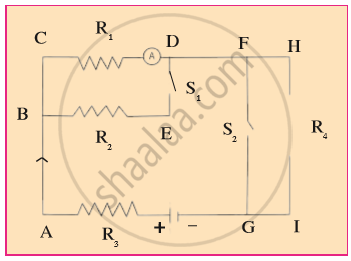a. Both S1 and S2 are closed.
b. Both S1 and S2 are open.
c.  S1 is closed but S2 is open.

Exercise | Q 8 | Page 45

Three resistances x1, x2 and x3 are connected in a circuit in different ways.  x is the effective resistance. The properties observed for these different ways of connecting x1, x2 and x3 are given below. Write the way in which they are connected in each case. (I-current, V-potential difference, x-effective resistance)

a.  Current I flows through x1, x2 and x3
b.  x is larger than x1, x2 and x3
c. x is smaller than x1, x2 and x3
d. The potential difference across x1, x2 and x3 is the same
e.  x = x1 + x2 + x3
f. x = 1/(1/X_1 + 1/X_2 + 1/X_3)

Exercise | Q 9.1 | Page 45

Solve the following problem.

The resistance of a 1m long nichrome wire is 6 W. If we reduce the length of the wire to 70 cm. what will its resistance be?              (Answer : 4.2  W)

Exercise | Q 9.2 | Page 45

Solve the following problem.
When two resistors are connected in series, their effective resistance is 80 W. When they are connected in parallel, their effective resistance is 20 W. What are the values of the two resistances?    (Answer : 40 W , 40 W)

Exercise | Q 9.3 | Page 45

Solve the following problem.
If a charge of 420 C flows through a conducting wire in 5 minutes what is the value of the current?                             (Answer : 1.4 A)

## Chapter 3: Current Electricity

Exercise## Balbharati solutions for Maharashtra state board SSC 9th Standard Science and Technology chapter 3 - Current Electricity

Balbharati solutions for Maharashtra state board SSC 9th Standard Science and Technology chapter 3 (Current Electricity) include all questions with solution and detail explanation. This will clear students doubts about any question and improve application skills while preparing for board exams. The detailed, step-by-step solutions will help you understand the concepts better and clear your confusions, if any. Shaalaa.com has the Maharashtra State Board Maharashtra state board SSC 9th Standard Science and Technology solutions in a manner that help students grasp basic concepts better and faster.

Further, we at Shaalaa.com provide such solutions so that students can prepare for written exams. Balbharati textbook solutions can be a core help for self-study and acts as a perfect self-help guidance for students.

Concepts covered in Maharashtra state board SSC 9th Standard Science and Technology chapter 3 Current Electricity are Potential Difference, Free Electrons, Electric Current, Resistance and Ohm'S Law, Electric Circuit, Conductors and Insulators, Experimental Proof of Ohm'S Law, System of Resistors and Effective Resistance.

Using Balbharati Class 9 solutions Current Electricity exercise by students are an easy way to prepare for the exams, as they involve solutions arranged chapter-wise also page wise. The questions involved in Balbharati Solutions are important questions that can be asked in the final exam. Maximum students of Maharashtra State Board Class 9 prefer Balbharati Textbook Solutions to score more in exam.

Get the free view of chapter 3 Current Electricity Class 9 extra questions for Maharashtra state board SSC 9th Standard Science and Technology and can use Shaalaa.com to keep it handy for your exam preparation